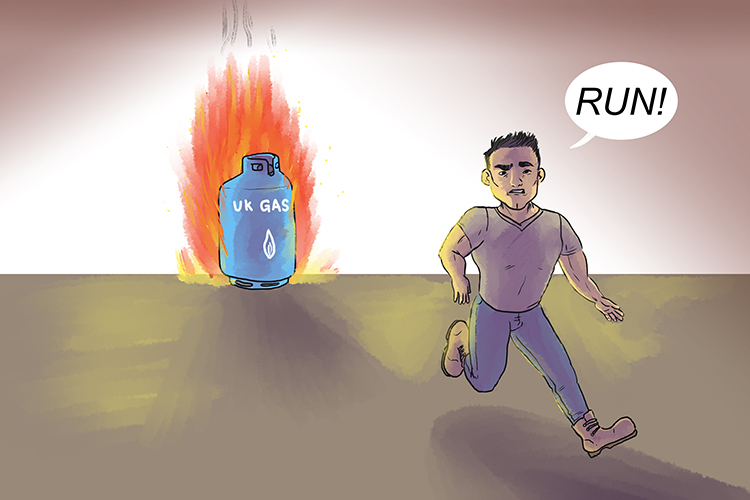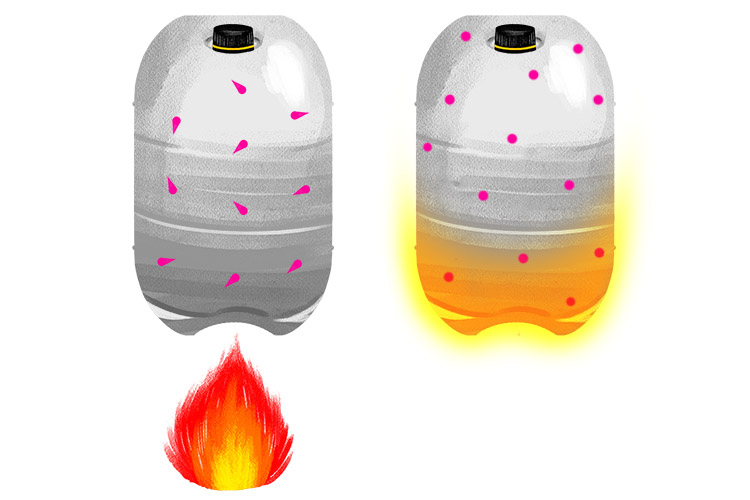# Gay-Lussac's Law In Use

We know in Gay-Lussac's law

P_1/T_1=P_2/T_2

NOTE:

Volume remains constant

Also you know that if you put a sealed container in a fire it will make the pressure inside the canister increase.This is Gay-Lussac's law

P_1 and T_1 refer to the pressure and temperature before you throw the container in the fire and  P_2 and T_2 refer to the pressure and temperature after you throw the container in the fire.

Increasing the temperature of the gas increases the pressure in the container.Increasing the temperature of a gas speeds up the molecules and they have more energy (kinetic energy) to hit and push the side of the container. The molecules will exert more pressure.

NOTE 1:

The standard unit used to measure pressure is Pa (Pascals)

The standard unit used to measure temperature is K (Kelvin)

NOTE 2:

You can use other units for pressure such as pounds per square inch or mm of mercury (mm Hg) or atm (atmospheres) as long as you use the same units either side of the equation but you must use Kelvin for temperature.

NOTE 3:

Never forget to convert temperature to Kelvin

Temperature in Kelvin = Temperature in degrees Celsius (\ ^(circ)C)+273

You must always use temperature measured in Kelvin in any gas law equation.

Examples

1. A 30\ \l sample of nitrogen inside a rigid, metal container at 20^circC is placed inside an oven whose temperature is 50^circC. The pressure inside the container at 20^circC was at 3.00  atm. What is the pressure of the nitrogen after its temperature is increased to 50^circC ?

1. A 30\ \l sample of nitrogen inside a rigid, metal container at 20^circC is placed inside an oven whose temperature is 50^circC. The pressure inside the container at 20^circC was at 3.00  atm. What is the pressure of the nitrogen after its temperature is increased to 50^circC ?

P_1/T_1=P_2/T_2

P_1=3atm

P_2=?

T_1=20^circC+273=293K

T_2=50^circC+273=323K

Therefore 3/293=P_2/323

P_2=(3xx323)/293

P_2=3.31atm (to three significant figures)

2. If a gas in a closed container is pressurized from 15 atmospheres to 16 atmospheres and its original temperature was 25^circC, what would the final temperature of the gas be in degrees Celsius?

2. If a gas in a closed container is pressurized from 15 atmospheres to 16 atmospheres and its original temperature was 25^circC, what would the final temperature of the gas be in degrees Celsius?

P_1/T_1=P_2/T_2

P_1=15atm

P_2=16atm

T_1=25^circC+273=298K

T_2=?

Therefore 15/298=16/T_2

T_2=(16xx298)/15

T_2=317.9K

But don't forget to convert back from Kelvin to degrees Celsius

Therefore T_2=317.9-273=44.9^circC

3. The temperature of a sample of gas in a steel container at 30\ \kPa is increased from -100^circC to 1.00xx10^3\ \^circC. What is the final pressure inside the tank.

3. The temperature of a sample of gas in a steel container at 30\ \kPa is increased from -100^circC to 1.00xx10^3\ \^circC. What is the final pressure inside the tank.

P_1/T_1=P_2/T_2

P_1=30\ \kPa

P_2=?

T_1=-100^circC+273=173K

T_2=1.00xx10^3\ \^circC=1000^circC+273=1273K

Therefore P_2=(P_1xxT_2)/T_1

P_2=(30xx1273)/173

P_2=221\ \kPa to three significant figures